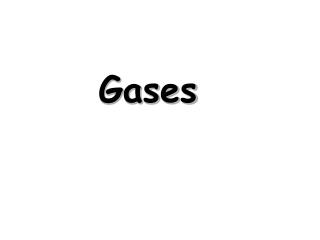DownloadDownload PresentationGases

# Gases

Download Presentation## Gases

- - - - - - - - - - - - - - - - - - - - - - - - - - - E N D - - - - - - - - - - - - - - - - - - - - - - - - - - -
##### Presentation Transcript

1. Gases

2. Ideal Gases Ideal gases are imaginary gases that perfectly fit all of the assumptions of the kinetic molecular theory. • Gases consist of tiny particles that are far apart • relative to their size. • Collisions between gas particles and between • particles and the walls of the container are • elastic collisions • No kinetic energy is lost in elastic collisions

3. Ideal Gases (continued) • Gas particles are in constant, rapid motion. They • therefore possess kinetic energy, the energy of • motion • There are no forces of attraction between gas • particles • The average kinetic energy of gas particles • depends on temperature, not on the identity • of the particle.

4. Gases expand to fill their containers Gases are fluid – they flow Gases have low density 1/1000 the density of the equivalent liquid or solid Gases are compressible Gases effuse and diffuse The Nature of Gases

5. Is caused by the collisions of molecules with the walls of a container 101.3 kPa = 1 atm = 760 mm Hg = 760 torr Pressure

6. Measuring Pressure The first device for measuring atmospheric pressure was developed by Evangelista Torricelli during the 17th century. The device was called a “barometer” • Baro = weight • Meter = measure

7. An Early Barometer The normal pressure due to the atmosphere at sea level can support a column of mercury that is 760 mm high.

8. P = 1 atmosphere, 760 torr T = 0°C, 273 Kelvins The molar volume of an ideal gas is 22.42 liters at STP Standard Temperature and Pressure“STP”

9. Converting Celsius to Kelvin Gas law problems involving temperature require that the temperature be in KELVINS! Kelvins = C + 273 °C = Kelvins - 273

10. Boyle’s Law Pressure is inversely proportional to volume when temperature is held constant.

11. Charles’s Law • The volume of a gas is directly proportional to temperature, and extrapolates to zero at zero Kelvin. (P = constant)

12. Gay Lussac’s Law The pressure and temperature of a gas are directly related, provided that the volume remains constant.

13. Combined Gas Law The combined gas law expresses the relationship between pressure, volume and temperature of a fixed amount of gas. Boyle’s law, Gay-Lussac’s law, and Charles’ law are all derived from this by holding a variable constant.

14. For a gas at constant temperature and pressure, the volume is directly proportional to the number of moles of gas (at low pressures). V=an a = proportionality constant V = volume of the gas n = number of moles of gas Avogadro’s Law

15. PV = nRT P = pressure in atm V = volume in liters n = moles R = proportionality constant = 0.08206 L atm/ mol·K = 8.314 L kPa/ mol T = temperature in Kelvins Ideal Gas Law Holds closely at P < 1 atm

16. Standard Molar Volume Equal volumes of all gases at the same temperature and pressure contain the same number of molecules. - Amedeo Avogadro

17. Gas Density … so at STP…

18. Gas Stoichiometry #1 If reactants and products are at the same conditions of temperature and pressure, then mole ratios of gases are also volume ratios. 3 H2(g) + N2(g)  2NH3(g) 3 moles H2 + 1 mole N2  2 moles NH3 3 liters H2 + 1 liter N2  2 liters NH3

19. Gas Stoichiometry #2 How many liters of ammonia can be produced when 12 liters of hydrogen react with an excess of nitrogen? 3 H2(g) + N2(g)  2NH3(g) 12 L H2 2 L NH3 = L NH3 8.0 3 L H2

20. Gas Stoichiometry #3 How many liters of oxygen gas, at STP, can be collected from the complete decomposition of 50.0 grams of potassium chlorate? 2 KClO3(s)  2 KCl(s) + 3 O2(g) 50.0 g KClO3 1 mol KClO3 3 mol O2 22.4 L O2 122.55 g KClO3 2 mol KClO3 1 mol O2 = L O2 13.7

21. Gas Stoichiometry #4 How many liters of oxygen gas, at 37.0C and 0.930 atmospheres, can be collected from the complete decomposition of 50.0 grams of potassium chlorate? 2 KClO3(s)  2 KCl(s) + 3 O2(g) 50.0 g KClO3 1 mol KClO3 3 mol O2 = “n” mol O2 0.612 mol O2 122.55 g KClO3 2 mol KClO3 = 16.7 L

22. For a mixture of gases in a container, PTotal = P1 + P2 + P3 + . . . Dalton’s Law of Partial Pressures This is particularly useful in calculating the pressure of gases collected over water.

23. Particles of matter are ALWAYS in motion Volume of individual particles is  zero. Collisions of particles with container walls cause pressure exerted by gas. Particles exert no forces on each other. Average kinetic energy µ Kelvin temperature of a gas. Kinetic Molecular Theory

24. Diffusion Diffusion: describes the mixing of gases. Therateof diffusion is the rate of gas mixing.

25. Effusion: describes the passage of gas into an evacuated chamber. Effusion

26. Graham’s LawRates of Effusion and Diffusion Effusion: Diffusion: